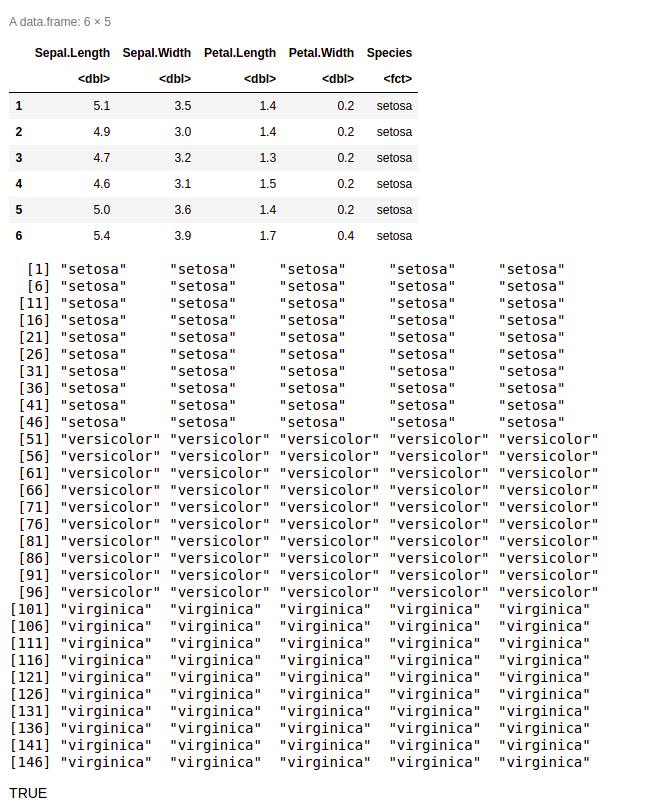Related Articles

# Convert dataframe column to vector in R

• Last Updated : 21 Apr, 2021

In this article, we will discuss how to convert a DataFrame column to vector in R Programming Language. To extract a single vector from a data frame in R Programming language, as.vector() function can be used.

Syntax: as.vector( data_frame\$column_name )

Here,

• data_frame is the name of the data frame
• column_name is the column to be extracted

Given below are some implementations for this.

Example 1:

## R

 `# creating dataframe ``std.data <- ``data.frame``(std_id = ``c ``(1:5), ``                       ``std_name = ``c``(``"Ram"``,``"Shayam"``,``"Mohan"``,``                                    ``"Kailash"``,``"Aditya"``),``                       ``marks = ``c``(95,96,95,85,80)``                      ``)`` ` `# extracting vector from``# dataframe column std_name``name.vec <- ``as.vector``(std.data\$std_name)``print``(name.vec) `

Output:

 “Ram”     “Shayam”  “Mohan”   “Kailash” “Aditya”

We can now examine whether the returned column is a vector or not, by passing it to the function is.vector() which returns a Boolean value i.e. either true or false.

Example 2:

We will extract the Species column from the well-known data frame Iris using as.vector( ) function and print it. We will also check whether the returned column is a vector or not.

## R

 `df <- iris`` ` `# print the data frame``head``(df)`` ` `# extracting vector from``# dataframe column Species``name.vec <- ``as.vector``(df\$Species)`` ` `print``(name.vec)`` ` `# returns Boolean value``is.vector``(name.vec) `

Output:My Personal Notes arrow_drop_up